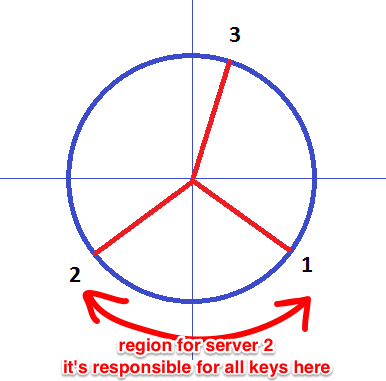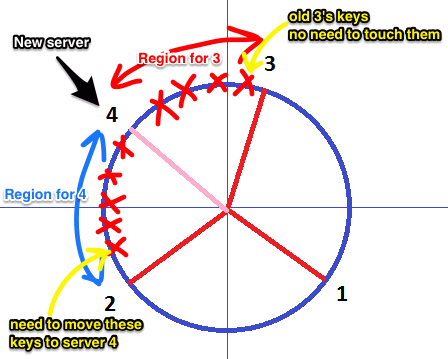# ML Wiki

## Consistent Hashing

To scale incrementally, Distributed Databases need a mechanism to dynamically partition over a set of nodes. Consistent Hashing is one of them: it allows to distribute load across several nodes.

### "Regular" hashing

• need assign $M$ data keys to $N$ servers
• assign each key to server number $k \text{ mod } N$

What happens if we increase a number of serves from $N$ to $2N$? Every existent key will have to be remapped.

### Consistent Hashing approach

• In consistent hashing a hash function is viewed as a ring: largest hash values wrap around to smallest
• The ring is divided onto $N$ regions ($N$ - number of servers)
• Each server has its own key region (its "position" on the ring)
• $\Rightarrow$ adding or removing a node affects only direct neighborsfor example

• the key region for 2nd server is the area between 1 and 2
• and only 2 is responsible for keys in that region

Suppose we want to add a new server

• we just pick some area
• and divide it on 2 parts
• and then assign the new server one of these two
• the keys that happen to be in that region are moved to the new serverSo routing is simple in this schema:

• each server knows the key range which it manages
• so we can route the request to the server that is closes to the key we're looking for

### Virtual Nodes

There are some challenges with this basic approach

• heterogeneity is performance is assumed (that is, we assume that all the servers have same performance)

A variant of Consistent Hashing algorithm addresses this issue:

• instead of mapping a single node to the ring,
• each node gets multiple points there
• so each node has several virtual nodes

A virtual node looks like a single node, but it refers to the real node.